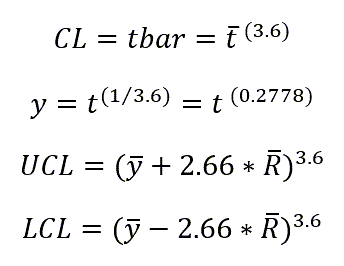# t Chart Formulas

### t Charts help evaluate time between rare events:

• wrong site or wrong patient surgeries
• cardiac arrests
• patient falls

Just count the time or number of units between events.
Transform the time into into a more normal distribution (y).
Calculate the range (R) between events.
Then use y and R to calculate the UCL and LCL (if any).

###t = time between rare occurrences

y = time transformed

R = moving range of y's

### What does "Transformed Time" refer to?

When using the t-Chart, note that it is calculating a rare occurrence, which is then modeled by the Poisson distribution, then the times between occurrences will be exponentially distributed. The exponential distribution is highly skewed, so plotting the times would result in a control chart that is difficult to interpret. The exponential can be transformed to a symmetric Weibull distribution by raising the time measure to the 1/3.6 = 0.2777 power or [y = t 0.27777].

The t Chart is just one of the tools included in the QI Macros for Excel SPC Software for Excel.

### Why Choose QI Macros Over Other Control Chart Software?### Affordable

• Only \$299 USD - less with quantity discounts
• No annual subscription fees
• Free technical support### Easy to Use

• Works right in Excel
• Create a chart in seconds
• Easy to customize & share charts### Proven and Trusted

• More than 100,000 users
• In More than 80 countries
• Five Star CNET Rating - Virus free